# Turning things upside-downElectronics guide > Analogue integrated circuits > Turning things upside-down

Earlier, we saw how the op-amp has two inputs, which we called non-inverting and inverting inputs. Now if we used the non-inverting input of the op-amp to make a non-inverting amplifier (Figure 9.5) then it makes sense that the inverting input of the op-amp may be used to make an inverting amplifier, as shown in Figure 9.7.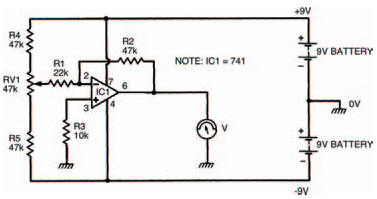Figure 9.7 Our next experimental circuit: an inverting amplifier

Build the circuit up on your breadboard, as shown in the breadboard layout of Figure 9.8. In a similar way to the last circuit; now take a number of voltage measurements of input and output voltages, to confirm that this really is an inverting amplifier. Remember that whatever polarity the input voltage is, the output voltage should be the opposite, so you’ll have to change round the meter leads to suit.

Hint:

One final point — the input to the op-amp circuit is classed as the connection between resistor R1 and the preset resistor, not the inverting input terminal at pin 2 — you’ll get some peculiar results if you try to measure the input voltage at pin 2!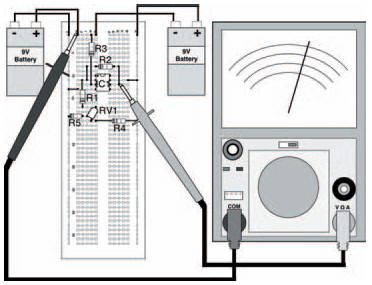Figure 9.8 The breadboard layout for the circuit in Figure 9.7

Tabulate your results in Table 9.3. The results from my circuit are given in Table 9.4, for reference. You’ll see that the circuit does invert the voltage, but also amplifies it by two at the same time.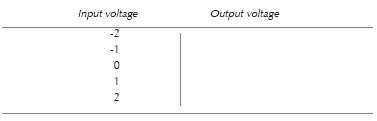Table 9.3 To show the results of your measurements

Like the non-inverting amplifier, the inverting amplifier’s gain is determined by resistance values, given this time by the expression: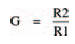so that the gain may once again be altered to suit a required application, just by changing a resistor.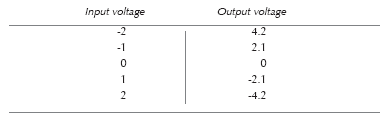Table 9.4 Shows the results of my measurements

<< Building on blocs